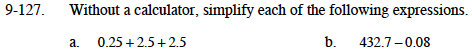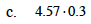### Home > MC1 > Chapter 9 > Lesson 9.3.1 > Problem9-127

9-127.Try doing this one without making any calculations on paper. What is 2.5 + 2.5?

$\text{Here, you are subtracting }\frac{8}{100} \text{ from }432\frac{70}{100}.$

Which are like parts?$\text{It might be useful to think of this as }\left(4\frac{57}{100}\right)\left(\frac{3}{10}\right)\text{ or }\left(\frac{457}{100}\right)\left(\frac{3}{10}\right)$
$\frac{(457)(3)}{1000}=\frac{1371}{1000}=1\frac{371}{1000}=1.371$